# BASIC ENGINEERING SCIENCE BASIC ENGINEERING SCIENCE ELECTRIC CHARGE

• Slides: 29BASIC ENGINEERING SCIENCEBASIC ENGINEERING SCIENCE ELECTRIC CHARGE, FORCE AND FIELD üElectric Charge üElectrostatic Charging üElectric Force üElectric Field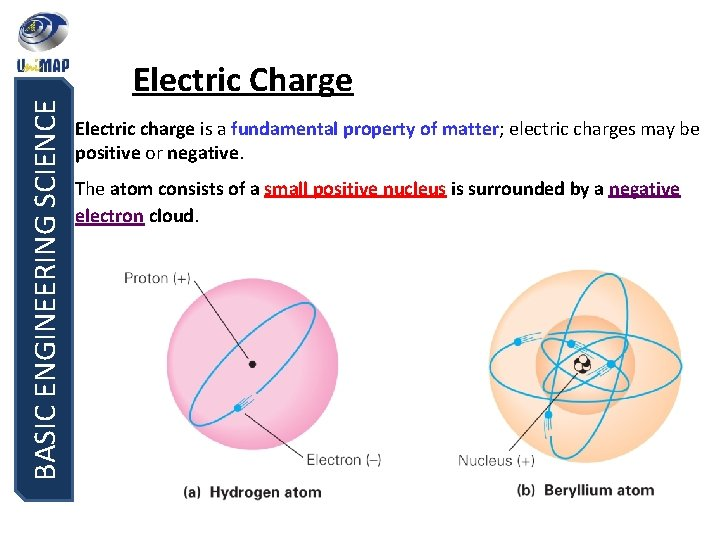BASIC ENGINEERING SCIENCE Electric Charge Electric charge is a fundamental property of matter; electric charges may be positive or negative. The atom consists of a small positive nucleus is surrounded by a negative electron cloud.BASIC ENGINEERING SCIENCE Electric Charge -Is an intrinsic characteristic of the fundamental particles making up those objects; that is, it is a characteristic that automatically accompanies those particles wherever they exist. Charges with the same electrical sign repel each other; and charges with opposite electrical signs attract each other.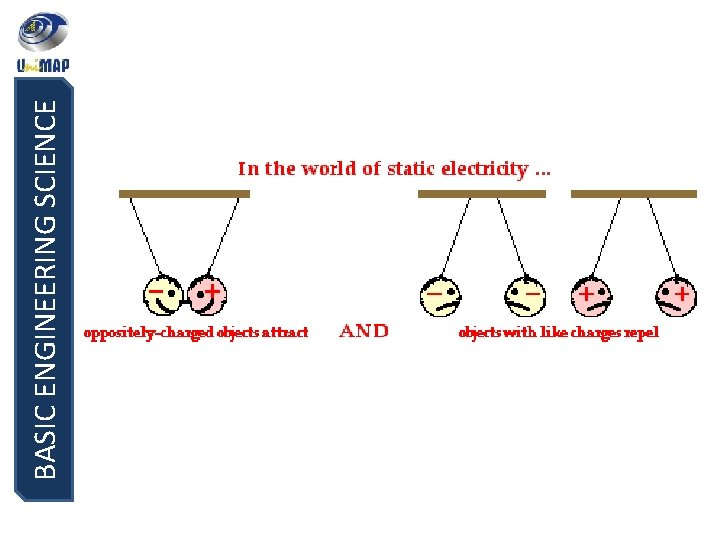BASIC ENGINEERING SCIENCE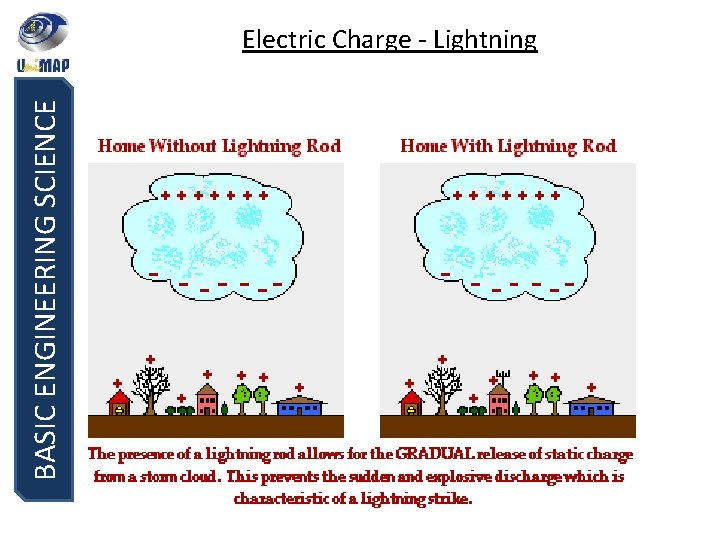BASIC ENGINEERING SCIENCE Electric Charge - Lightning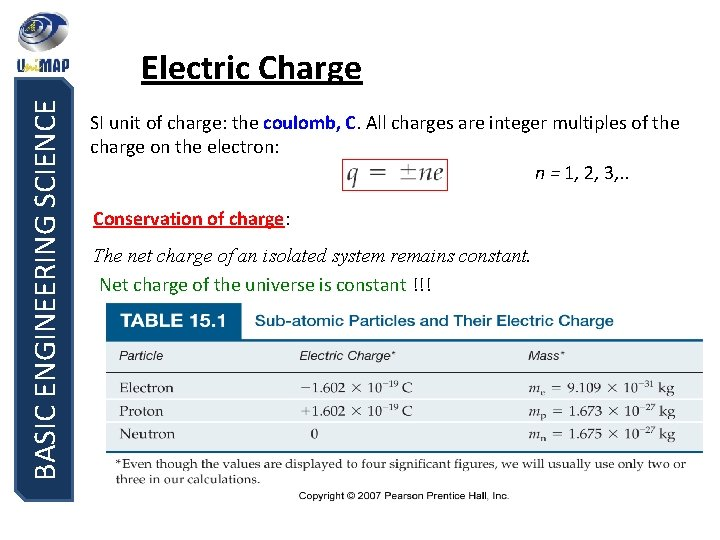BASIC ENGINEERING SCIENCE Electric Charge SI unit of charge: the coulomb, C. All charges are integer multiples of the charge on the electron: n = 1, 2, 3, . . Conservation of charge: The net charge of an isolated system remains constant. Net charge of the universe is constant !!!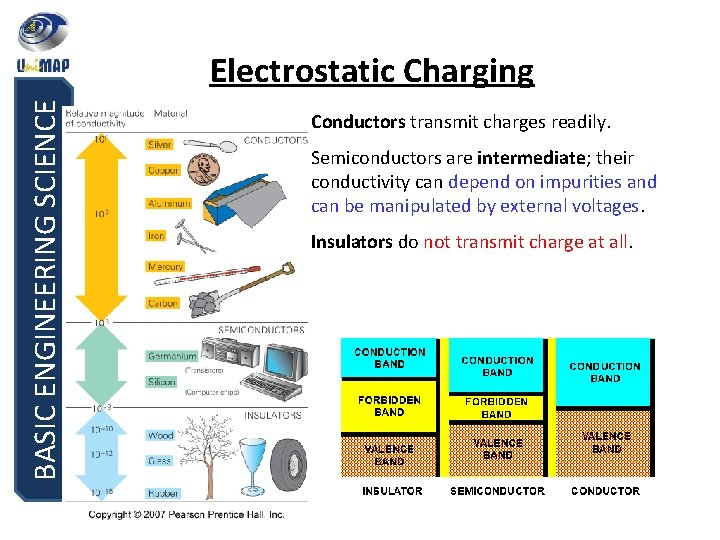BASIC ENGINEERING SCIENCE Electrostatic Charging Conductors transmit charges readily. Semiconductors are intermediate; their conductivity can depend on impurities and can be manipulated by external voltages. Insulators do not transmit charge at all.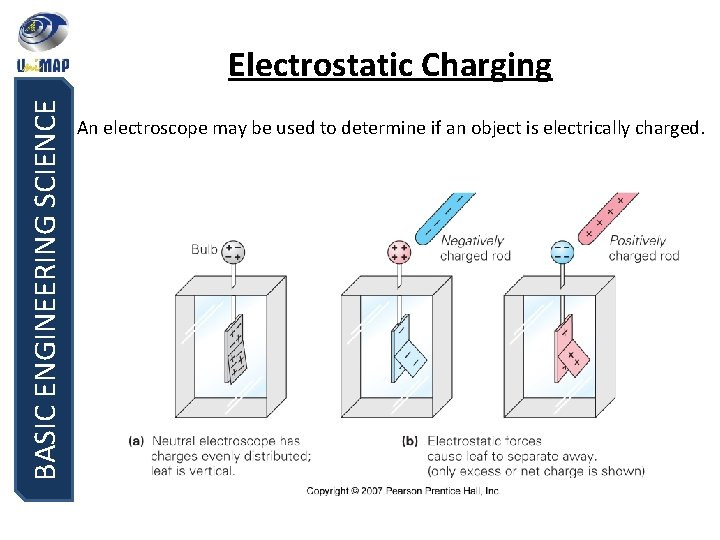BASIC ENGINEERING SCIENCE Electrostatic Charging An electroscope may be used to determine if an object is electrically charged.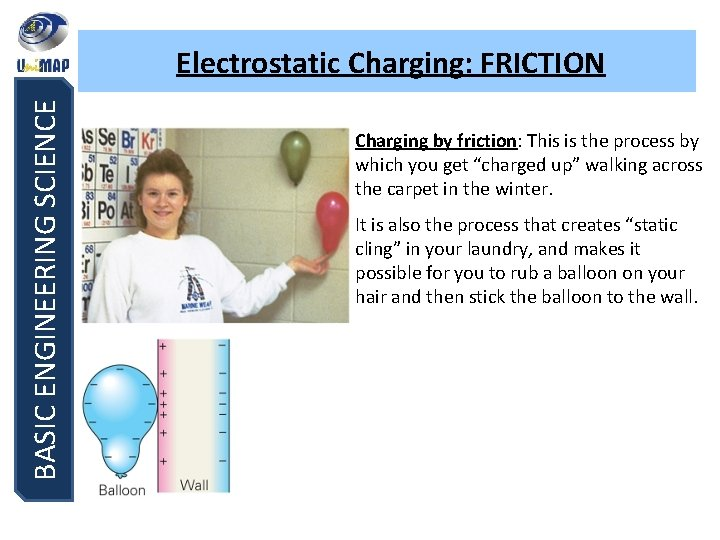BASIC ENGINEERING SCIENCE Electrostatic Charging: FRICTION Charging by friction: This is the process by which you get “charged up” walking across the carpet in the winter. It is also the process that creates “static cling” in your laundry, and makes it possible for you to rub a balloon on your hair and then stick the balloon to the wall.BASIC ENGINEERING SCIENCE Electrostatic Charging: CONDUCTION An electroscope can be given a net charge by conduction – when it is touched with a charged object, the excess charges flow freely onto the electroscope.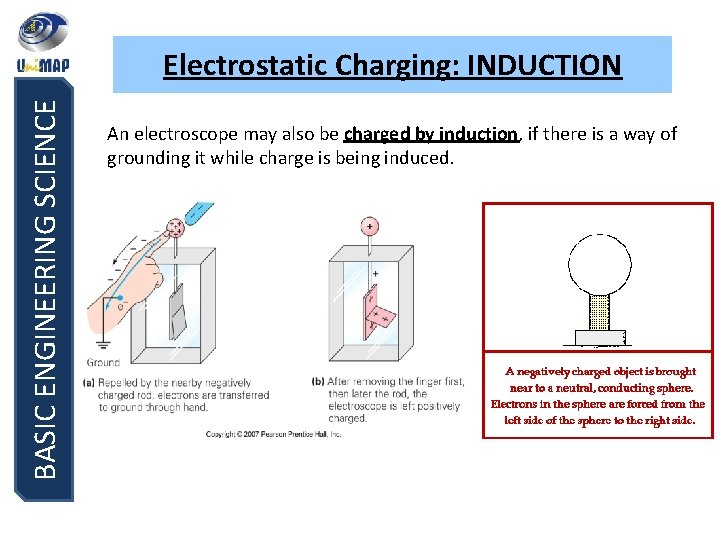BASIC ENGINEERING SCIENCE Electrostatic Charging: INDUCTION An electroscope may also be charged by induction, if there is a way of grounding it while charge is being induced.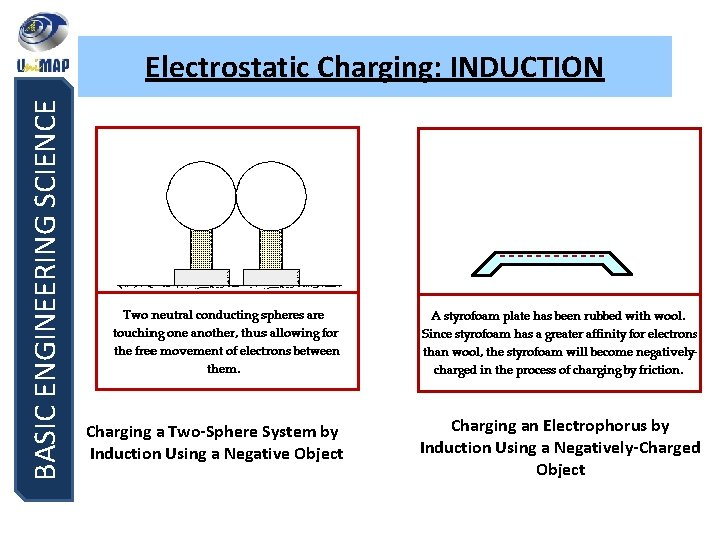BASIC ENGINEERING SCIENCE Electrostatic Charging: INDUCTION Charging a Two-Sphere System by Induction Using a Negative Object Charging an Electrophorus by Induction Using a Negatively-Charged Object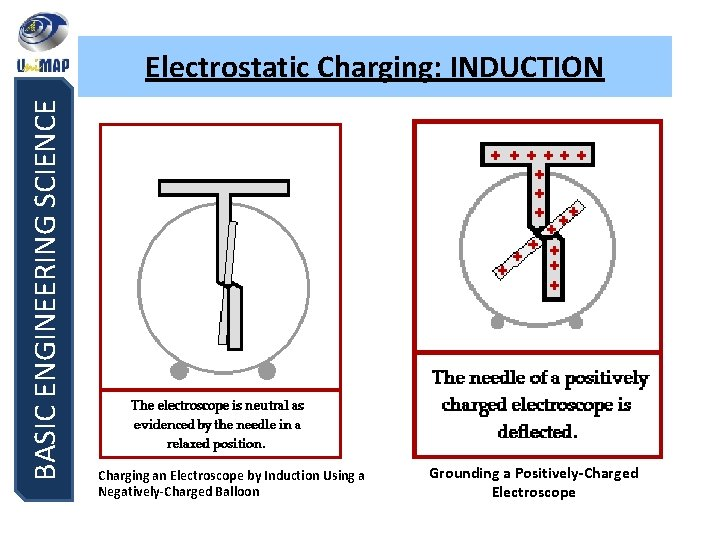BASIC ENGINEERING SCIENCE Electrostatic Charging: INDUCTION Charging an Electroscope by Induction Using a Negatively-Charged Balloon Grounding a Positively-Charged ElectroscopeBASIC ENGINEERING SCIENCE Electrostatic Charging: POLARIZATION Charge may also be moved within an object – without changing its net charge – through a process called polarization. (charge separation by polarization)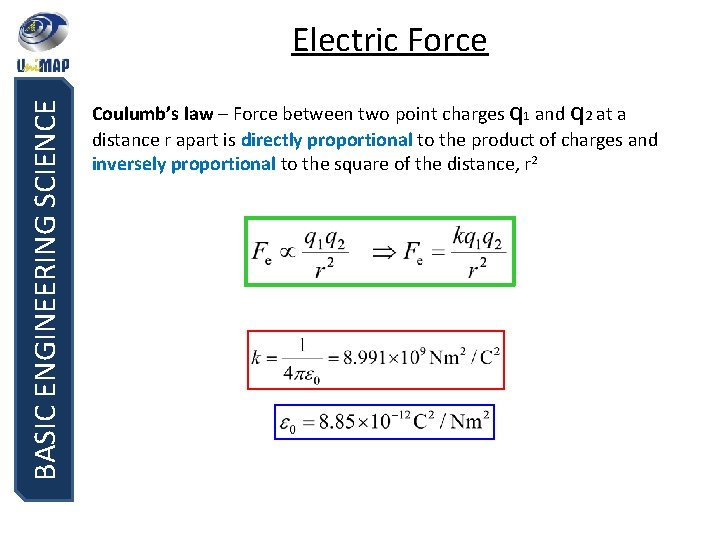BASIC ENGINEERING SCIENCE Electric Force Coulumb’s law – Force between two point charges q 1 and q 2 at a distance r apart is directly proportional to the product of charges and inversely proportional to the square of the distance, r 2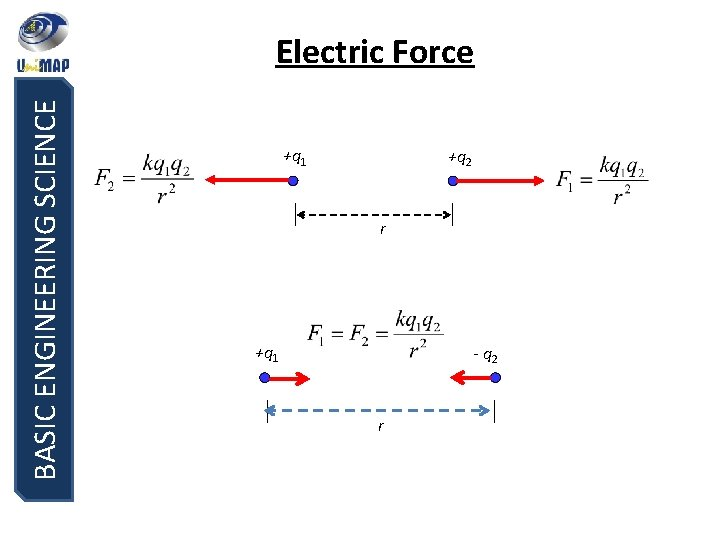BASIC ENGINEERING SCIENCE Electric Force +q 1 +q 2 r +q 1 - q 2 rBASIC ENGINEERING SCIENCE Electric Force If there are multiple point charges, the force vectors must be added to get the net force.BASIC ENGINEERING SCIENCE Example 1: Two point charges of -1. 0 n. C and +2. 0 n. C are separated by a distance of 0. 3 m, what is the electric force on each particle? q 1 = -1 n. C F 21 F 12 0. 3 m Solution: q 2 = +2 n. C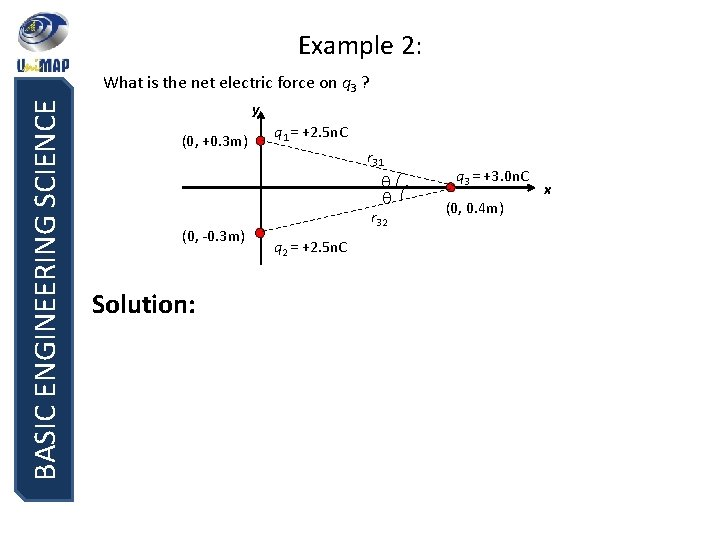Example 2: BASIC ENGINEERING SCIENCE What is the net electric force on q 3 ? y (0, +0. 3 m) q 1 = +2. 5 n. C r 31 (0, -0. 3 m) Solution: r 32 q 2 = +2. 5 n. C q 3 = +3. 0 n. C (0, 0. 4 m) x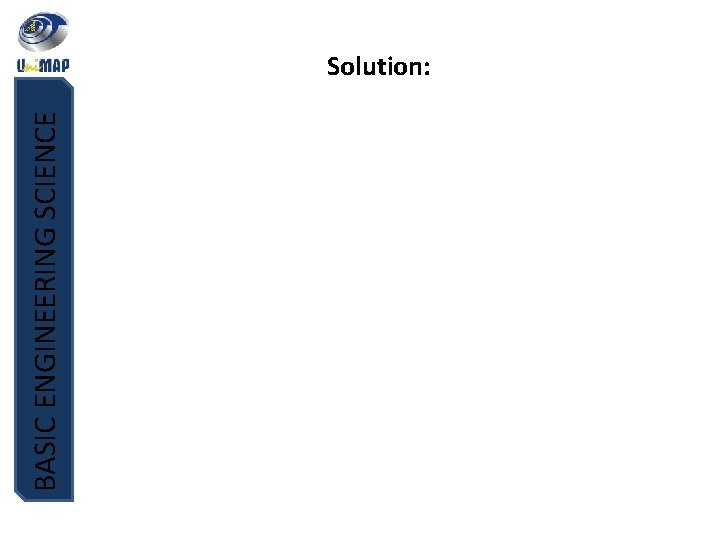BASIC ENGINEERING SCIENCE Solution: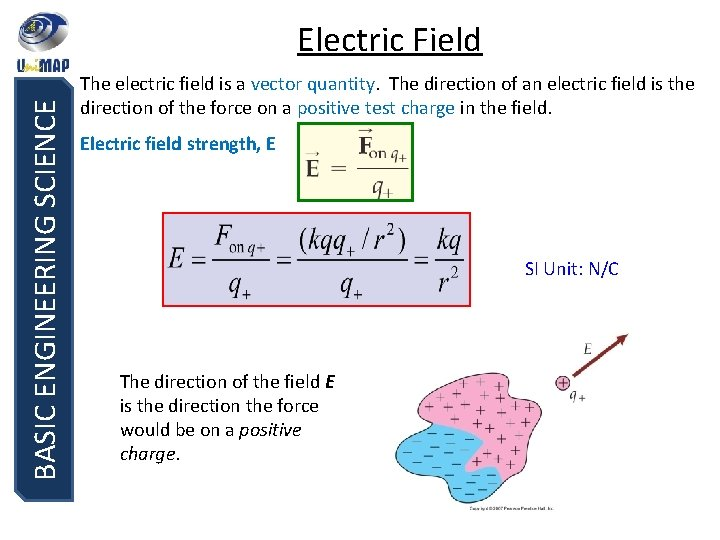BASIC ENGINEERING SCIENCE Electric Field The electric field is a vector quantity. The direction of an electric field is the direction of the force on a positive test charge in the field. Electric field strength, E SI Unit: N/C The direction of the field E is the direction the force would be on a positive charge.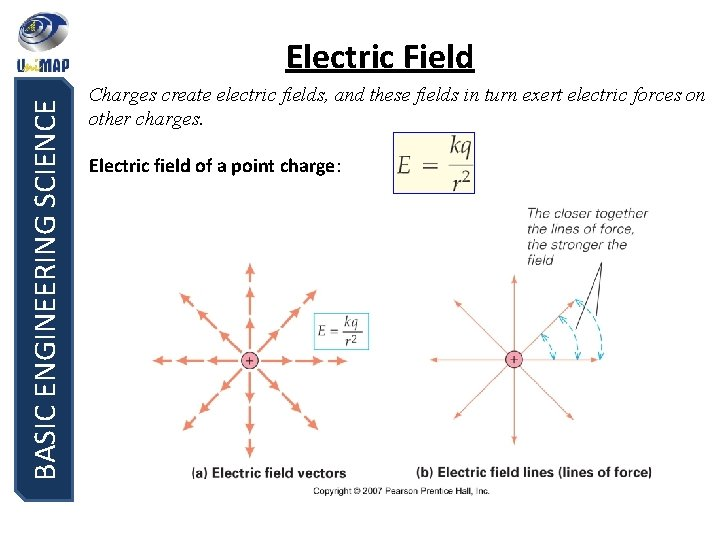BASIC ENGINEERING SCIENCE Electric Field Charges create electric fields, and these fields in turn exert electric forces on other charges. Electric field of a point charge:BASIC ENGINEERING SCIENCE Example 2: Find the magnitude and direction of the electric field strength at the point P due to the point charges at A and B, as shown in the figure P 10 cm A + +2µC 10 cm -8µC BBASIC ENGINEERING SCIENCE Solution:BASIC ENGINEERING SCIENCE Exercise y q 1 q 3 d d 30 o x q 2 1. Fig. above shows three particles with charge q 1 = +2 Q, q 2 = -2 Q, and q 3 = -4 Q, each a distance d=30 cm, from the origin. What net electric field E is produced at the origin? y -8μC s +2μC s P s +12μC x 2. Find the electric field at point P due to the charges shown where s = 50 cm.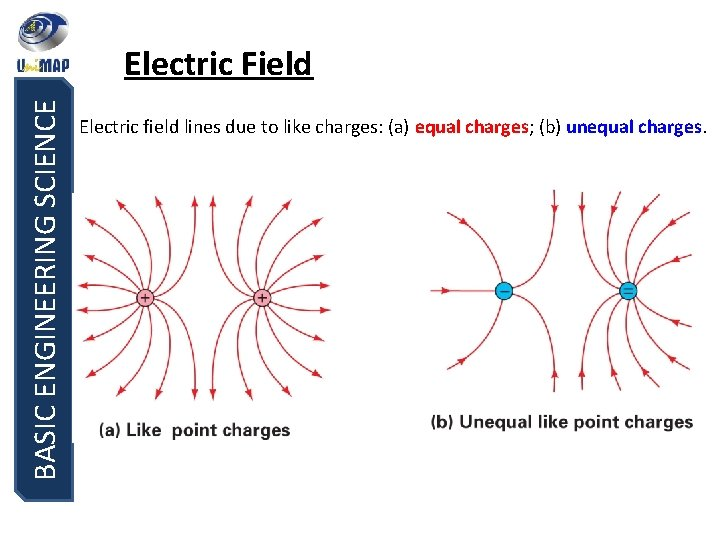BASIC ENGINEERING SCIENCE Electric Field Electric field lines due to like charges: (a) equal charges; (b) unequal charges.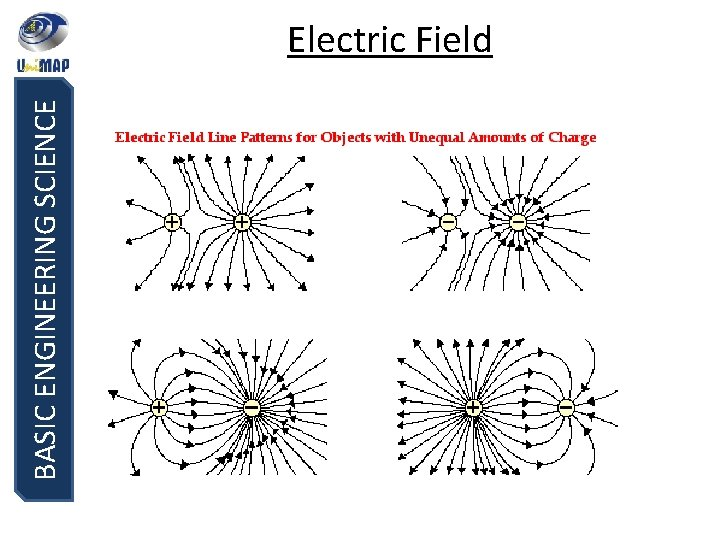BASIC ENGINEERING SCIENCE Electric FieldBASIC ENGINEERING SCIENCE END OF CHAPTER 5